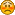# July Handicapping

Dawn
Battle Hill Farm

Days Handicapped: 8
Win: 63 x \$250 = \$15,750
Place: 44 x \$100 = \$4400
Show: 64 x \$50 = \$3200
Quinella: 16 x \$500 = \$8000
Trifecta: 7 x \$1000 = \$7000
Total: \$38,350

Southern Cross TC

Total Days Handicapped 1
Win: 6 x \$250 = \$1500
Place: 6 x \$100 = \$600
Show: 12 x \$50 = \$600
Quinella: 3 x \$500 = \$1500
Trifecta: 0 x \$1000 = \$0
Total: \$4,200

This Month
Days Handicapped = 7
Win: 70 x \$250 = \$17500
Place: 48 x \$100 = \$4800
Show: 59 x \$50 = \$2950
Quinella: 21 x \$500 = \$10500
Trifecta: 9 x \$1000 = \$9000
Total: \$44,750

Degs74 (Paul)
Dege Destroyers

Carisbrooke (LC)
Carisbrooke Stable

This Month
Number of Days Handicapped: 2
Wins: 9 x \$250 = \$2,250
Places: 6 x \$100 = \$600
Shows: 10 x \$50 = \$500
Quinella: 2 x \$500 = \$1,000
Trifecta: 6 x \$1,000 = \$6,000
Total: \$10,350

Days Handicapped: 1
Win: 12 x \$250 = \$3000
Place: 3 x \$100 = \$300
Show: 11 x \$50 = \$550
Quinella: 0 x \$500 = \$0
Trifecta: 2 x \$1000 = \$2000
Total: \$5850

Copper Hill Farm
Days Handicapped = 7

Win: 43 x \$250 = \$10750
Place: 55 x \$100 = \$5500
Show: 45 x \$50 = \$2250
Quinella: 10 x \$500 = \$5000
Trifecta: 7 x \$1000 = \$7000
Total: \$30,500

Total Days - 6
Wins - \$9,750
Places - \$2,500
Shows - \$1,700
Quinellas - \$4,500
Trifectas - \$7,000
Total - \$25,450

Drew
Railbird Racing

Days Handicapped: 3
Win: 25 x \$250 = \$6,250
Place: 13 x \$100 = \$1,300
Show: 22 x \$50 = \$1,100
Quinella: 7 x \$500 = \$3,500
Trifecta: 3 x \$1000 = \$3,000
Total: \$15,150

Name:Lilly/Rainee TBS
Days Handicapped: 3
Win: 13 x \$250 = \$3,250
Place: 9 x \$100 = \$900
Show: 14 x \$50 = \$700
Quinella: 4 x \$500 = \$2,000
Trifecta: 1 x \$1000 = \$1,000
Total:\$7,850

Bethany
Windsor Arms

6 days

26 wins=6500
18 place=1800
16 shows=800
7 exacta=3500
1 trifecta=1000
total=13,600

The above amounts have been awarded, unless you ignored the rules and didn’t post your stable name.

Uhm, I’m sorry I’m so late… If I can’t get the money, I understand.LeAnn
Freedom Acres

Number of Days Handicapped: 4
Wins: 20 x \$250 = \$5,000
Places: 26 x \$100 = \$2,600
Shows: 33 x \$50 = \$1,650
Quinella: 8 x \$500 = \$4,000
Trifecta: 6 x \$1,000 = \$6,000
Total: \$19,250